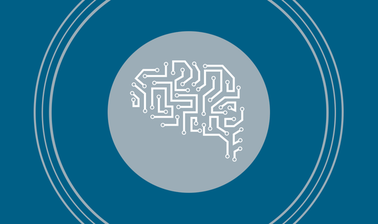# Machine Learning

This course focuses on core algorithmic and statistical concepts in machine learning. Topics include pattern recognition, PAC learning, overfitting, decision trees, classification, linear regression, logistic regression, gradient descent, feature projection, dimensionality reduction, maximum likelihood, Bayesian methods, and neural networks.This course is archived
Estimated 12 weeks
8–12 hours per week
Instructor-paced
Instructor-led on a course schedule
Free

Tools from machine learning are now ubiquitous in the sciences with applications in engineering, computer vision, and biology, among others. This class introduces the fundamental mathematical models, algorithms, and statistical tools needed to perform core tasks in machine learning. Applications of these ideas are illustrated using programming examples on various data sets.

Topics include pattern recognition, PAC learning, overfitting, decision trees, classification, linear regression, logistic regression, gradient descent, feature projection, dimensionality reduction, maximum likelihood, Bayesian methods, and neural networks.

### At a glance

• Institution: UTAustinX
• Subject: Computer Science
• Prerequisites:

Linear Algebra, Probability, Experience programming in Python

• Language: English
• Video Transcript: English

# What you'll learn

Skip What you'll learn

○ Techniques for supervised learning including classification and regression.
○ Algorithms for unsupervised learning including feature extraction.
○ Statistical methods for interpreting models generated by learning algorithms.

# Syllabus

Skip Syllabus

Mistake Bounded Learning (1 week)
Decision Trees; PAC Learning (1 week)
Cross Validation; VC Dimension; Perceptron (1 week)
Linear Regression; Gradient Descent (1 week)
Boosting (.5 week)
PCA; SVD (1.5 weeks)
Maximum likelihood estimation (1 week)
Bayesian inference (1 week)
K-means and EM (1-1.5 week)
Multivariate models and graphical models (1-1.5 week)
Neural networks; generative adversarial networks (GAN) (1-1.5 weeks)